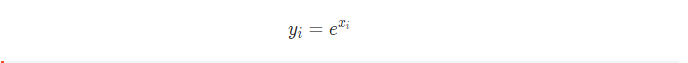Related Articles
Python – PyTorch exp() method
• Last Updated : 26 May, 2020

PyTorch `torch.exp()` method returns a new tensor after getting the exponent of the elements of the input tensor.Syntax: `torch.exp(input, out=None)`

Arguments

• input: This is input tensor.
• out: The output tensor.

Return: It returns a Tensor.

Let’s see this concept with the help of few examples:
Example 1:

 `# Importing the PyTorch library ``import` `torch ``   ` `# A constant tensor of size n``a ``=` `torch.randn(``6``)``print``(a)`` ` `# Applying the exp function and ``# storing the result in 'out'``out ``=` `torch.exp(a)``print``(out)`

Output:

```1.0532
-1.9300
0.6392
-0.7519
0.9133
0.3998
[torch.FloatTensor of size 6]
2.8667
0.1451
1.8949
0.4715
2.4925
1.4915
[torch.FloatTensor of size 6]

```

Example 2:

 `# Importing the PyTorch library ``import` `torch ``   ` `# A constant tensor of size n``a ``=` `torch.FloatTensor([``1``, ``4``, ``6``, ``3``])``print``(a)`` ` `# Applying the exp function and ``# storing the result in 'out'``out ``=` `torch.exp(a)``print``(out)`

Output:

``` 1
4
6
3
[torch.FloatTensor of size 4]
2.7183
54.5981
403.4288
20.0855
[torch.FloatTensor of size 4]
```

Attention geek! Strengthen your foundations with the Python Programming Foundation Course and learn the basics.

To begin with, your interview preparations Enhance your Data Structures concepts with the Python DS Course.

My Personal Notes arrow_drop_up